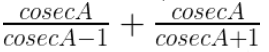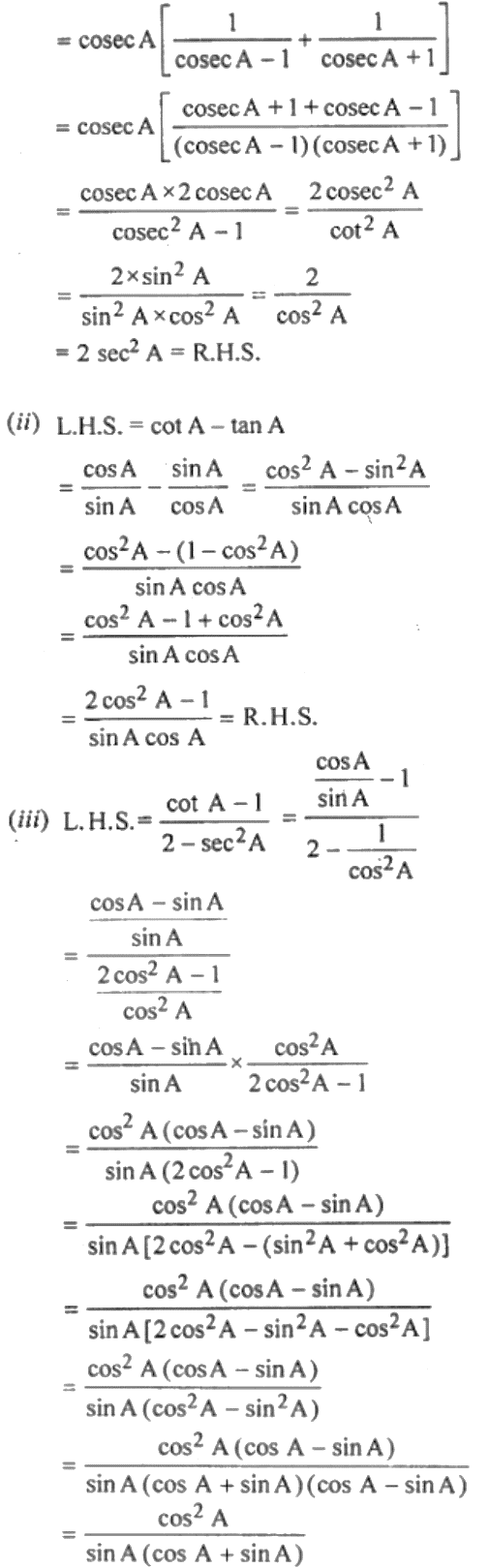Guru

# (i) cosec A/ (cosec A – 1) + cosec A/ (cosec A + 1) = 2 sec2 A (ii) cot A – tan A = (2cos2 A – 1)/ (sin A – cos A) (iii) (cot A – 1)/ (2 – sec2 A) = cot A/ (1 + tan A)

• 0

This ques has been asked in previous year question paper of class 10 and is the very important ques from the Book- ML Aggarwal
Board- ICSE
Publication- Avichal
Chapter- Trigonometric Identities
Chapter number-18
Number of questions- 35

(i) cosec A/ (cosec A – 1) + cosec A/ (cosec A + 1) = 2 sec2 A

(ii) cot A – tan A = (2cos2 A – 1)/ (sin A – cos A)

(iii) (cot A – 1)/ (2 – sec2 A) = cot A/ (1 + tan A)

Class 10, chapter 18th, trigonometric identities

Share